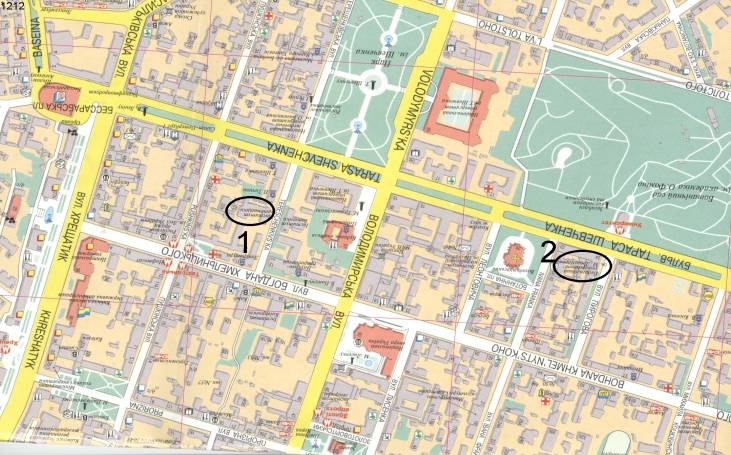# UKRAINIAN MATHEMATICAL CONGRESS 2001Legend:      1 – Institute of Mathematics;

2 – National Pedagogical Drahomanov University

## Section locations:

Institute of Mathematics:

Lecture hall:

305 (conference hall) – Section 3: Differential equations and nonlinear oscillations;

101 (library) – Section 5: Mathematical Physics;

208 – Section 10: Theory of Approximations and Harmonic Analysis.

National Pedagogical Drahomanov University:

Lecture hall:

448 – Section 1: Algebra and Theory of Numbers;

320 – Section 2: Dynamical Systems;

419 – Section 4: Complex Analysis and Potential Theory;

319 – Section 6: Teaching Methods and History of Mathematics;

462 – Section 8: Computational Mathematics and Mathematical Problems of Mechanics;

451, 431 – Section 9: Probability Theory and Mathematical Statistics;

337,340-342 – Section 11: International Conference on Functional Analysis;

461 – Section 12: Topology and Geometry;

456 – Section 13: Mathematical Theory of Control.